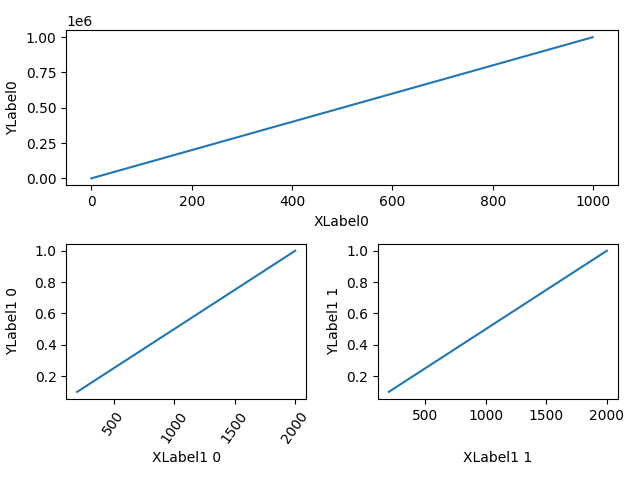# Aligning Labels¶

Aligning xlabel and ylabel using Figure.align_xlabels and Figure.align_ylabels

Figure.align_labels wraps these two functions.

Note that the xlabel "XLabel1 1" would normally be much closer to the x-axis, and "YLabel1 0" would be much closer to the y-axis of their respective axes.import matplotlib.pyplot as plt
import numpy as np
import matplotlib.gridspec as gridspec

fig = plt.figure(tight_layout=True)
gs = gridspec.GridSpec(2, 2)

ax = fig.add_subplot(gs[0, :])
ax.plot(np.arange(0, 1e6, 1000))
ax.set_ylabel('YLabel0')
ax.set_xlabel('XLabel0')

for i in range(2):
ax = fig.add_subplot(gs[1, i])
ax.plot(np.arange(1., 0., -0.1) * 2000., np.arange(1., 0., -0.1))
ax.set_ylabel('YLabel1 %d' % i)
ax.set_xlabel('XLabel1 %d' % i)
if i == 0:
for tick in ax.get_xticklabels():
tick.set_rotation(55)
fig.align_labels()  # same as fig.align_xlabels(); fig.align_ylabels()

plt.show()


Keywords: matplotlib code example, codex, python plot, pyplot Gallery generated by Sphinx-Gallery